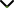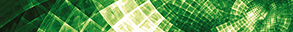Recent Advances in Fixed Point Theory in Abstract Spaces

Publishing date
01 Dec 2021
Status
Closed
30 Jul 2021

Guest Editors

1Muş Alparslan University, Mus, Turkey

2University of Management and Technology, Lahore, Pakistan

3University of Banja Luka, Banja Luka, Bosnia and Herzegovina

This issue is now closed for submissions.
More articles will be published in the near future.

Recent Advances in Fixed Point Theory in Abstract Spaces

This issue is now closed for submissions.
More articles will be published in the near future.

DescriptionFixed Point Theory is an integrative theory that provides discernment and significant tools for the solution of certain problems in several areas of interest in mathematics as well as other disciplines, such as biology, engineering, physics, computer sciences, data sciences, economics, etc.

The existence and uniqueness of solutions for linear and nonlinear problems is commonly denoted as the fixed point problems Tx=x, where T is self-operator in an abstract space X such as the existence and uniqueness of solutions to elliptic partial differential equations, and more recently the existence of answer sets in logic programming. The situation gets more complicated when self-operators are replaced with nonself-operators T:A → B, where A and B are nonempty and disjoint subsets of X, it is clear that no fixed point will exist for nonself-operators. In this situation, the concept and importance of Approximate Fixed Point are highlighted.

The objective of this Special Issue is to highlight advancements in fixed point and approximate fixed point theory in abstract spaces (Banach spaces, locally convex spaces, metric spaces, fuzzy metric spaces, etc.) and their applications to the solvability of nonlinear operator equations, which include ordinary differential equations, partial differential equations, functional differential equations, integral equations, integrodifferential equations, fractional differential equations, difference equations, etc. The solvability of those equations is usually investigated in specific function spaces. The choice of the appropriate fixed point theorem and the use the specific properties of the underlying function space can have a big impact on the solvability of those equations. Original research and review articles are welcome.

Potential topics include but are not limited to the following:

• Fixed point and approximate fixed point theorems in Banach spaces (for the weak and the strong topology)
• Fixed point and approximate fixed point theorems in Banach algebras
• Fixed point and approximate fixed point theorems in locally convex spaces
• Fixed point and approximate fixed point theorems in function spaces
• Fixed point and approximate fixed point theorems in fuzzy metric spaces
• Operator equations in function spaces
• Operator inclusions in function spaces
• Evolution equations in function spaces
• Stability of functional equations related to fixed point theory
• Nonlinear operator theory and applicationsJournal metrics
Acceptance rate22%
Submission to final decision43 days
Acceptance to publication32 days
CiteScore0.500
Journal Citation Indicator0.690
Impact Factor0.971Author guidelinesEditorial boardDatabases and indexing

Article of the Year Award: Outstanding research contributions of 2020, as selected by our Chief Editors. Read the winning articles.